/

### Function Description

Returns,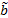, the weighted mean absolute deviation versus the median of a series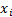(for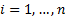), calculated as follows: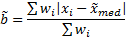(where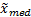is the weighted median of the series, see MnWeightedMedian)

See also MnWeightedMeanAbsDevVsMean which provides another common way of measuring mean absolute deviation. However, the mean absolute deviation is minimised if it is measured relative to the median of the series rather than relative to its mean.

See Weighted Moments and Cumulants for further details and explanation of terms in this formula. If the weights are equal then returns the same as MnMeanAbsDevVsMedian.

Contents | Prev | Next